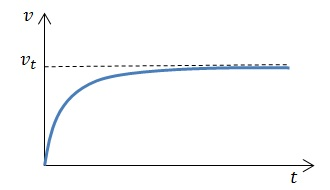# How to Calculate Air Resistance of a Falling Object

Whenever objects move relative to the air, the objects experience a resistive force$\vec{F_{r}}$ which is in the opposite direction to the body’s velocity relative to the air. This resistive force is called air resistance. For instance, consider a falling sheet of paper. Relative to the air, the paper is moving downwards, and so there will be an upward resistive force on the paper.

## How to Calculate Air Resistance of a Falling Object when the Object Falls Slowly in Air

For objects which move slowly relative to the air (such as falling dust particles), the resistive force is directly proportional to the object’s velocity$\vec{v}$ relative to air. So, we can write:$\vec{F_{r}} = -b\vec{v}$

The value of$b$ depends on the shape and size of the body.

Let us consider what happens to a body as it falls from rest at a low speed through the air. Initially, the body’s velocity relative to the air is 0 and so there is no air resistance. As the body speeds up under the resultant downwards force, the air resistance also increases in proportion.Eventually, the body reaches a speed where the body’s weight is exactly balanced by the air resistance. Here, the body has reached terminal velocity$v_{T}$. The variation of velocity with time can be shown on a graph, as follows:It can be shown that the terminal velocity for these cases is given by$v_{t} = \frac{mg}{b}$.

Example:

Suppose a pollen grain with a mass of 3.8×10-14 kg is falling through air. If the value of the constant$b =$ 4.0×10-11 kg s-1, find the terminal velocity.

Since$v_{t} = \frac{mg}{b}$,$v_{t} = \frac{3.8\times 10^{-14}\times9.81}{4\times10^{-11}} = 9.3\times10^{-3} \textrm m$

Note: In reality, the calculation is not so simple, with many other factors also coming into play. However, for the sake of this example, we have assumed that the only factors which affect the pollen grain’s fall are gravity and air resistance, and the air resistance is also assumed to be directly proportional to the grain’s velocity.

## How to Calculate Air Resistance of a Falling Object when the Object Falls Fast in Air

Objects falling faster in the air (for example, skydivers) cause considerable amounts of turbulence, and consequently the air resistance experienced by them is much greater. For these objects, the air resistance is directly proportional to the square of the object’s velocity relative to air. The air resistance for these cases is given by:$F_r = \frac{1}{2}C_{d}\rho Av^2$

Here,$C_{d} =$ is drag coefficient,$\rho =$ is the density of air (typically about 1.2 kg m-3), and$A =$ is the effective cross section of the body perpendicular to the direction of the body’s velocity. Typically,$C_{d}$ takes values between 0.1 and 2.

The terminal velocity for objects moving fast in air can be given by$v_{t} = \sqrt{\frac{2mg}{C_{d}\rho A}}$.

Example:

A golf ball falling in air has a drag coefficient of 0.26. Given that it has an effective cross-sectional area of 1.4×10-3 m2, find the air resistance on the ball when the ball is moving at a speed of 20 m s-1.

We have$F_r = \frac{1}{2}C_{d}\rho Av^2$

Then,$F_r = \frac{1}{2} \times 0.26 \times 1.2 \times 1.4 \times 10^{-3}\times 20^2 = 0.087 \textup{ N}$